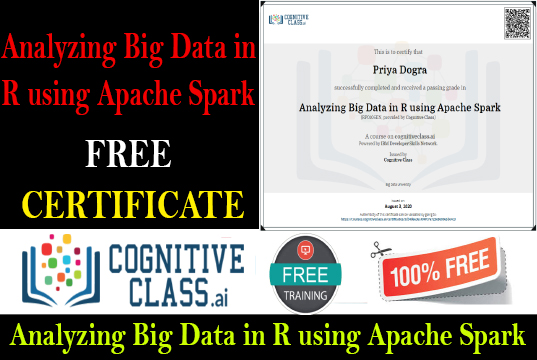Home Cognitive Class Analyzing Big Data in R using Apache Spark Cognitive Class Answers

# Analyzing Big Data in R using Apache Spark Cognitive Class Answers

0## Get Certificate: Analyzing Big Data in R using Apache Spark

Analyzing Big Data in R using Apache Spark

Module 1: Introduction to SparkR

Question 1: What shells are available for running SparkR??

• Spark-shell
• SparkSQL shell
• SparkR shell
• RSpark shell
• None of the options is correct

Question 2: What is the entry point into SparkR?

• SRContext
• SparkContext
• RContext
• SQLContext

Question 3: When would you need to call sparkR.init?

• using the R shell
• using the SR-shell
• using the SparkR shell
• using the Spark-shell

Module 2: Data manipulation in SparkR

Question 1: dataframes make use of Spark RDDs

• False
• True

Question 2: You need read.df to create dataframes from data sources?

• True
• False

Question 3: What does the groupBy function output??

• A AggregateOrder object
• A GroupedData object
• A OrderBy object
• A GroupBy object

Module3: Machine learning in SparkR

Question 1: What is the goal of MLlib?

• Integration of machine learning into SparkSQL
• To make practical machine learning scalable and easy
• Visualization of Machine Learning in SparkR
• Provide a development workbench for machine learning
• All of the options are correct

Question 2: What would you use to create plots? check all that apply

• pandas
• Multiplot
• Ggplot2
• matplotlib
• all of the above are correct

Question 3: Spark MLlib is a module of Apache Spark

• False
• True

Analyzing Big Data in R using Apache Spark Final Exam Answers Cognitive Class

Question 1: Which of these are NOT characteristics of Spark R?

• it supports distributed machine learning
• it provides a distributed data frame implementation
• is a cluster computing framework
• a light-weight front end to use Apache Spark from R
• None of the options is correct

Question 2: True or false? The client connection to the Spark execution environment is created by the shell for users using Spark:

• True
• False

Question 3: Which of the following are not features of Spark SQL?

• performs extra optimizations
• works with RDDs
• is a distributed SQL engine
• is a Spark module for structured data processing
• None of the options is correct

Question 4: True or false? Select returns a SparkR dataframe:

• False
• True

Question 5: SparkR defines the following aggregation functions:

• sumDistinct
• Sum
• count
• min
• All of the options are correct

Question 6: We can use SparkR sql function using the sqlContext as follows:

• head(sql(sqlContext, “SELECT * FROM cars WHERE cyl > 6”))
• SparkR:head(sql(sqlContext, “SELECT * FROM cars WHERE cyl > 6”))
• SparkR::head(sql(sqlContext, “SELECT * FROM cars WHERE cyl > 6”))
• SparkR(head(sql(sqlContext, “SELECT * FROM cars WHERE cyl > 6”)))
• None of the options is correct

Question 7: Which of the following are pipeline components?

• Transformers
• Estimators
• Pipeline
• Parameter
• All of the options are correct

Question 8: Which of the following is NOT one of the steps in implementing a GLM in SparkR:

• Evaluate the model
• Train the model
• Implement model